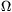Középiskolai Matematikai és Fizikai Lapok
Informatika rovattal
 Már regisztráltál? Új vendég vagy?# Exercises and problems in Physics December 1998

## New experimental problem:

m. 202. Measure the fractions of incident light reflected, transmitted and absorbed by a plastic ruler as a function of the angle of incidence. (6 points)

## New exercises:

FGy. 3204. The area of the point of a drawing-pin is approximately 0.1 mm2, while that of its head is 1.2 cm2. What pressure has to be exerted onto the head of the pin so that its point exerts a pressure one thousand times greater than the atmospheric pressure onto the drawing-board? What force is then applied? (3 points)

FGy. 3205. A consumer is connected to an accumulator across a series resistor. When the resistance of the series resistor is increased from 100to 150, the power output of the accumulator decreases by 25 %. By how many percents does the power consumption of the consumer with unchanged resistance decrease? (4 points)

FGy. 3206. A floating areometer is calibrated for densities between 800 kg/m3 and 2000 kg/m3, the distance between the signs corresponding to these is 28 cm. What is the distance between the bottom sign and the sign corresponding to 1400 kg/m3? (4 points)

FGy. 3207. There is a small, closed, evacuated tube with heat-insulating walls in a large container containing He gas. The pressure of the He is 105 Pa, its temperature is 300 K. The end of the small tube is opened for a little while and some He enters. What is the temperature of the He in the tube? (4 points)

## New problems:

FF. 3208. Estimate the masses of the atmospheres of the Earth and Venus. The pressure at the surface of Venus is 90 times greater than that at the surface of the Earth, the mean diameter of Venus is 5 % smaller, its density 10 % smaller than the respective data of the Earth. (4 points)

FF. 3209. There is 1 mole of He gas at a temperature of 0 oC in a vertically standing, 5 m-high, heat-insulated cylinder with a cross-section of 0.01 m2. A heat-insulating piston with a mass of 4 kg, moving without friction, is held at the top of the cylinder. The atmospheric pressure is 105 Pa. How deep does the piston sink when released? (4 points)

FF. 3210. In principle, both the Earth and the Moon could be charged electrically by shooting electrons from them into space. What would be the minimum work required to form an electrostatic repulsion strong enough to finally separate the Moon from the Earth? What would the majority of this work be used for? (5 points)

FF. 3211. A rod of mass m and resistance R is placed perpendicularly onto a pair of sufficiently long, parallel bars of negligible resistance, at distance l from each other in a horizontal plane. The rod can slide without friction parallelly to itself on the bars. One end of the pair of bars is open, the other is connected to a capacitor of capacitance C charged to p.d. U. The bars are in a homogeneous, vertical magnetic field of induction B. After connecting the capacitor to the bars, what is the final speed of the rod and what is the final p.d. across the capacitor? What should the mass of the rod be to allow the maximum kinetic energy? (5 points)

FF. 3212. White light is shone onto a 250 nm-thick soap membrane at an angle of incidence of 30o. What is the colour of the light reflected from the membrane? (5 points)